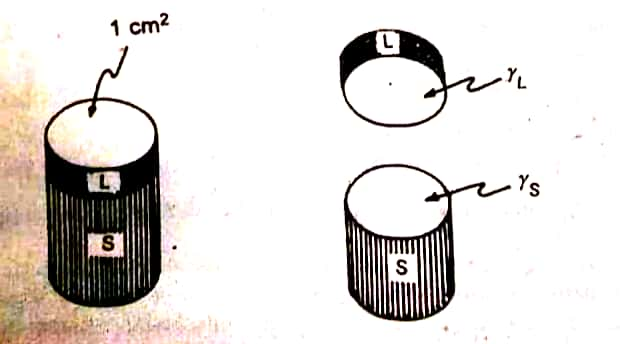HKT's Membership Quiz Contest is running 🏆 for Students. Hurry up. Click here to Participate

Spreading Coefficient Important Questions – Physical Pharmacy

Contents

Important Questions:
2. Give application of spreading coefficient.
3. Give derivation of spreading coefficient.
4. Why benzene spreads on water.
5. What are two way by which you can increase spreading coefficient of given organic substance.

Spreading: If a small quantity of an immiscible liquid is placed on the surface of another liquid, it will either spread as a film on surface of the other liquid or remain as a drop or lens. Which of the two applies generally depends on the achievement of a state of minimum free energy. The ability of one liquid to spread over another can be assessed in terms of spreading coefficient whose value should either be positive or zero for spreading to occur.
Spreading is particularly important for products meant for external application such as lotions, creams, etc. These should be able to spread freely and evenly on the skin.
In general spreading of a liquid on the surface of another occurs when the work of adhesion between the two liquids exceeds the works of cohesion between the molecule of each liquid.2. Give application of spreading coefficient.

When a drop of oleic acid is placed on the surface of water, it will spread as a film. If the spreading is spontaneous, the surface free energy of the systems is reduced. The principles of spreading coefficient find applications in pharmacy.

1. Absorption of medicament: When a cream or lotion is applied on the skin, it has to be spread on the affected area and permits absorption of medicament. Normally surfactants are added to the formulation to increase the polarity and the spreading coefficient.
2. Stabilization of emulsions: A blend of surfactants are mixed with an oil and its spreading on the water surface layer is evaluated. Based on the results, a suitable blend of surfactants is selected for the preparation of a stable emulsion.

3. Give derivation of spreading coefficient.

We are to follow these steps:
1. Calculate work of cohesion (Wc).
2. Calculate work of adhesion. (Wa)
3. Carry our subtraction of Wa – Wc to calculate S.
4. Write inference of positive or negative value of S.
Calculation for work of cohesion (Wc).
It is the energy required to separate the molecule of the spreading liquid, say oleic acid. There is no interfacial tension, when the liquid alone is considered. Cohesive forces alone are operating. When a hypothetical cylinder (cross sectional area of 1 sq. cm) is divided, two new surfaces are created.
Calculation for work of adhesion. (Wa)
It is the energy required to bring out the adhesion between the unlike molecules. The same amount of  energy is required even to break the adhesive forces between liquids. Now, consider the hypothetical cylinder of the sublayer liquid, ‘S’ present below the similar section of the spreading liquid, L. The work done is equal to:Representation of the work of adhesion involving the separation of sublayer (S: water) and an overlaying liquid (L: oleic acid)

Carry our subtraction of Wa – Wc to calculate S.
When spreading of liquid (oleic acid) occurs, when the adhesive forces (represented by Wa) are stronger than the cohesive forces (Wc). The spreading coefficient is obtained by equation:

4. Why benzene spreads on water.

For spreading organic liquid on water, the initial coefficient may be either positive or negative, but the final spreading coefficient is negative.
Solvent benzene spreads on water, because of stronger adhesive forces over the cohesive forces, though polar groups are absent.

5. What are two way by which you can increase spreading coefficient of given organic substance.

Spreading coefficient of a substance can be increased by:
1. the presence of polar functional group such as -COOH or -OH in the structure. Examples are propanoic acid (S = 45.8); ethyl alcohol (S = 50.4).
2. reducing the nonpolar chain length. Example are petrolatum (S = -13.4); oleic acid (S = 24.6).

×